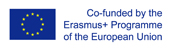This project has been funded with support from the European Commission.
This web site reflects the views only of the author, and the Commission cannot be held responsible for any use which may be made of the information contained therein.

Select language   >   IT EN RO PL LT

### Percentages_Exercises, Videos, Web Site/Portal

Subject taught
- Math

Type of Product
- Exercises
- Videos
- Web Site/Portal

Language Skills Developed
- Listening
- Speaking

Transferable/Scientific Skills Developed
The resource helps students to develop their self–directed learning and problem solving skills while learning to understand what a percentage is and how it can be used in real life situations.

Description
General aims:
The general aims of the resource are to explain what a percentage is, how to use it in real life situations and how to apply percentage calculations.
Linguistic aims:
To introduce key vocabulary: percentages, per cent, one hundredth, fractions, decimals, fractional parts; to use key vocabulary to explain what a percentage is and how it can be used in real life situations; to understand the content.
Subject specific aims:
To understand what the word percent means; to learn how it can be converted to a fraction; to understand what a “whole fraction” and an “improper fraction” means; to learn to convert any fraction into its decimal value; to re-write a percentage as a decimal number; To understand the importance and relevance of percentage calculations: pay rises, allowance rises, interest rates, discounts on sale items etc.; To analyse the examples of percentage calculations and to learn to work through percentage calculation problems.
Target group age: lower secondary school students (13-14 years old).
Level of competence in English (CEFR) B1/B1+
Time required to use the resource with the students depends on the aim of the lesson. It could be used for one or two lessons (45 – 90 min).
How to use it:
The teacher distributes copies of the worksheets to the students. Working individually, students revise the topic of percentages and get ready to answer the questions: What is a percentage? What is the formula for calculating percentages? After revising the theory, analysing examples of percentage calculations and analysing a common error when finding a percentage, students get other worksheets and work on percentage calculations individually.
To understand the calculations better, similar types of problems to those in the examples are solved in a series of three mini-lessons on Calculating with Percent.
Possible difficulties for the students: students whose knowledge of English is poor can face difficulties understanding the text.